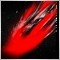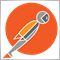# Using Array Values outside of a WebRequest Function12

Hello; I'm new to MQL4 and am attempting to retrieve values from a web request function to be used in a separate trading logic function but I cannot seem to get the data out of the local GetRequest function. There are 12 data points downloaded and I am using two of them, labeled p06 and p11, which are percent change values. These get refreshed with new values every 30 seconds. The data is being successfully imported via the WebRequest; I'm just not clear on the best approach for getting the values out of the function to be used elsewhere in the code. Any suggestions? Thank you!
```int OnInit()
{
//--- create timer
EventSetTimer(30); //30 secs

//---
return(INIT_SUCCEEDED);
}
//+------------------------------------------------------------------+
//| Expert deinitialization function                                 |
//+------------------------------------------------------------------+
void OnDeinit(const int reason)
{
//--- destroy timer
EventKillTimer();

}
//+------------------------------------------------------------------+
//| Expert tick function                                             |
//+------------------------------------------------------------------+
//void OnTick()
//  {
//---

//  }
//+------------------------------------------------------------------+
//| Timer function                                                   |
//+------------------------------------------------------------------+
void OnTimer()
{
//---

GetRequest();
}
//+------------------------------------------------------------------+
//| Web Request function                                             |
//+------------------------------------------------------------------+

void GetRequest()
string values;

//string referer = NULL;

int timeout = 15000; // 15 secs

char data[], result[];

int data_size;

string url = "http://localhost/analytics";

data_size = ArraySize(data);

int res;

if (res == -1) {
Print("Error in WebRequest. Error code = ", GetLastError());

} else {
ConvertResponse(CharArrayToString(result), values);

Print("p06 =>", values);
Print("p11 =>", values);

double p06 = values;
double p11 = values;

}

void ConvertResponse(string response, string& values[]) {
// parsing json object with this function...

Print("Response =>", response);

string value;
string items[];
ushort comma, dot;

response = StringSubstr(response, 1, StringLen(response) - 2);

comma = StringGetCharacter(",", 0);
dot = StringGetCharacter(":", 0);

int n = StringSplit(response, comma, items);

for(int i = 0; i < n; i++) {
string result[];

StringSplit(items[i], dot, result);

string key = result;

if(key == "\"p06\"") {
value = StringSubstr(result, 1, StringLen(result) - 2);
} else {
value = result;
}

values[i] = value;

}

}
```47934

```double GetRequest(int index // the index of the array which should be returned
){
string values;

//string referer = NULL;

int timeout = 15000; // 15 secs

char data[], result[];

int data_size;

string url = "http://localhost/analytics";

data_size = ArraySize(data);

int res;

if (res == -1) {
Print("Error in WebRequest. Error code = ", GetLastError());

return -1;

} else {
ConvertResponse(CharArrayToString(result), values);

Print("p06 =>", values);
Print("p11 =>", values);

return (double)values[index];
}
return -1;
}```

``` double p06 = GetRequest(5);
double p11 = GetRequest(10);```12

Pavel Shutovskiy:

Thank you!!! The code is now working successfully.2465

falconer:
Thank you!!! The code is now working successfully.

Don't actually use that code. I'm not sure if that was intended to troll you or not... but you do realize it's making two consecutive web request for the same data, right? Also, you shouldn't parse JSON like that because your code will fall apart the second a single char is out of place. Something like this is how you need to implement your code.

```#include <jason.mqh> // https://www.mql5.com/en/code/13663

bool getRequest(string &json_response)
{
char req_body[];
char response[];
int status_code =  WebRequest(
"GET",           // HTTP method
"http://localhost/analytics",              // URL
15000,          // timeout
req_body,          // the array of the HTTP message body
response,        // an array containing server response data
);
json_response = CharArrayToString(response);
return (status_code == 200);
}

void OnStart()
{
//string test;
//if (!getRequest(test))
//   return;
string test = "{\"data\":[1,2,3,4,5], \"error\": false}";
CJAVal json_parser;
json_parser.Deserialize(test);
printf("data=%d, data=%d, error=%s",
json_parser["data"].ToInt(),
json_parser["data"].ToInt(),
string(json_parser["error"].ToBool())
);
}```12

nicholi shen:

Don't actually use that code. I'm not sure if that was intended to troll you or not... but you do realize it's making two consecutive web request for the same data, right? Also, you shouldn't parse JSON like that because your code will fall apart the second a single char is out of place. Something like this is how you need to implement your code.

Thank you, I will test this today.